# Test: Vector Algebra- 1

## 25 Questions MCQ Test Mathematics For JEE | Test: Vector Algebra- 1

Description
Attempt Test: Vector Algebra- 1 | 25 questions in 25 minutes | Mock test for JEE preparation | Free important questions MCQ to study Mathematics For JEE for JEE Exam | Download free PDF with solutions
QUESTION: 1

### Vector has

Solution:

A vector has both magnitude as well as direction.

QUESTION: 2

### Correct form of distributive law is

Solution:

Distributive law is given by :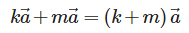QUESTION: 3

### Magnitude of the vector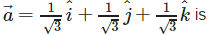Solution:

We have :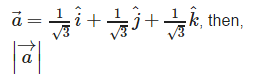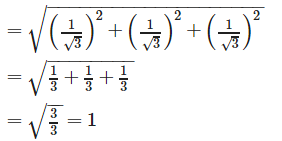QUESTION: 4

Find the unit vector in the direction of vector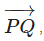where P and Q are the points (1, 2, 3) and (4, 5, 6), respectively

Solution: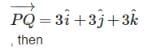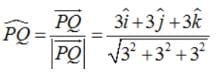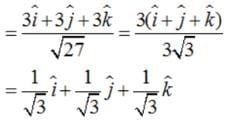QUESTION: 5

If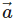is a non zero vector of magnitude ‘a’ and λ a non zero scalar, then λis a unit vector if

Solution:

λis a unit vector if and only ifis equal to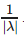QUESTION: 6

Find the values of x and y so that the vectors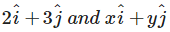are equal

Solution: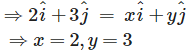QUESTION: 7

If P1(x1, y1, z1) and P2(x2, y2, z2) are any two points, then the vector joining P1 and P2is the vector P1P2. Magnitude of the vector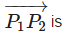Solution:

If P1(x1, y1, z1) and P2(x2, y2, z2) are any two points, then the vector joining P1 and P2is the vector P1P2, then ;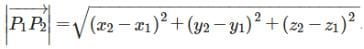QUESTION: 8

Find the scalar and vector components of the vector with initial point (2, 1) and terminal point (– 5, 7).

Solution:

The scalar and vector components of the vector with initial point (2, 1) and terminal point (– 5, 7) is given by : (- 5 – 2) i.e. – 7 and (7 – 1) i.e. 6. Therefore, the scalar components are – 7 and 6 .,and vector components are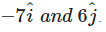QUESTION: 9

Find a vector in the direction of the vector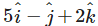which has a magnitude of 8 units

Solution: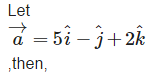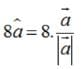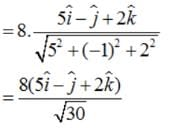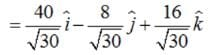QUESTION: 10

Find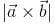, if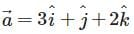and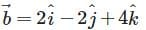Solution: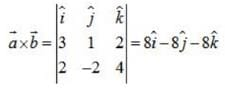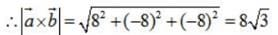QUESTION: 11

Direction angles are angles

Solution:

α,β,γ are the angles which the position vector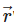makes with the positive x-axis ,y-axis and z-axis respectively are called direction angles.

QUESTION: 12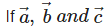are any three vectors then the correct expression for distributivity of scalar product over addition is

Solution:are any three vectors then the correct expression for distributivity of scalar product over addition is :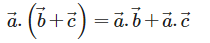QUESTION: 13

Find the values of x and y so that the vectorsSolution: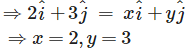QUESTION: 14

Find the direction cosines of the vector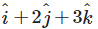Solution: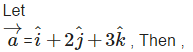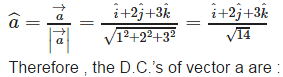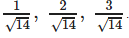QUESTION: 15

Find a unit vector perpendicular to each of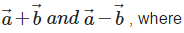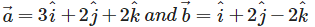Solution:

It is given that: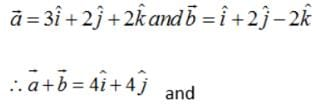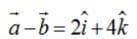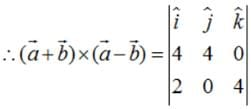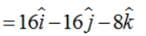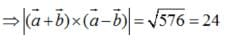Therefore, the unit vector perpendicular to both the vectors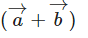and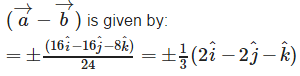QUESTION: 16

Direction cosines

Solution:

Cosines of the angles α,β,γ are called direction cosines.

QUESTION: 17

Magnitude of the vector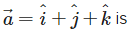Solution:

We have :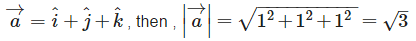QUESTION: 18

Find the sum of the vectors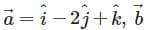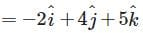and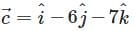Solution:

We have: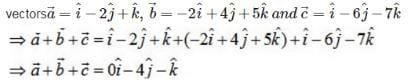QUESTION: 19

Find the direction cosines of the vector joining the points A(1, 2, –3) and B(–1, –2, 1), directed from A to B.

Solution: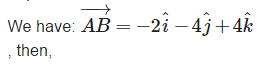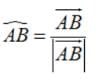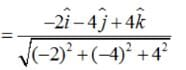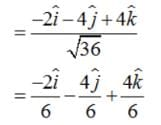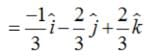Therefore, the D.C.’s of vector AB are given by: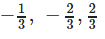QUESTION: 20

If a unit vector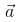makes angles π/3 with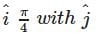and an acute angle θ with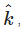then find θ

Solution: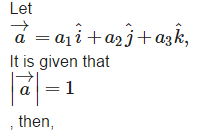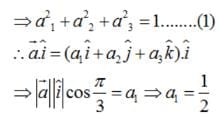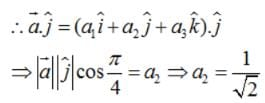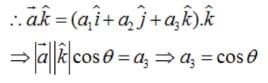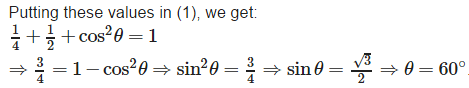QUESTION: 21

If l, m and n are direction cosines of the position vector OP the coordinates of P are

Solution:

If l , m and n are the direction cosines of vector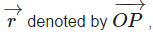then , the coordinates of point P are given by : lr ,mr and nr respectively.

QUESTION: 22

Unit vectors along the axes OX, OY and OZ are denoted by

Solution: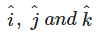represents the unit vectors along the co ordinate axis i.e. OX ,OY and OZ respectively.

QUESTION: 23

Write down a unit vector in XY-plane, making an angle of 30° with the positive direction of x-axis.

Solution: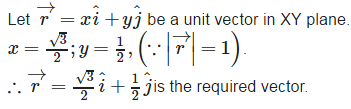QUESTION: 24

Find the angle between two vectors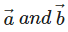with magnitudes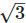and 2, respectively, having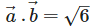Solution: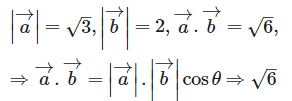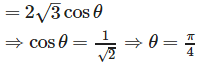QUESTION: 25

If a unit vector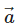makes angles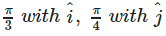and an acute angle θ with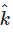, then the components ofare

Solution:

Let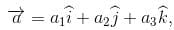It is given that left|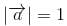, then ,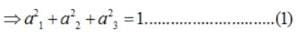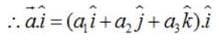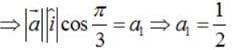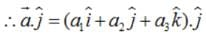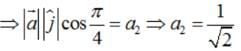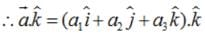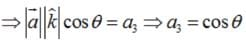Putting these values in (1) , we get :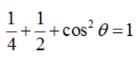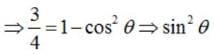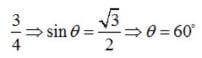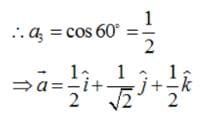Use Code STAYHOME200 and get INR 200 additional OFF Use Coupon Code